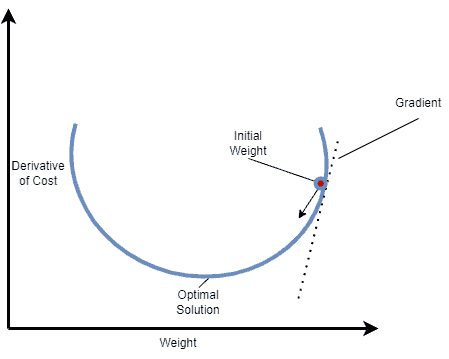## 1. Introduction

In this tutorial, we’ll talk about Gradient Descent (GD) and the different approaches that it includes. Gradient Descent is a widely used high-level machine learning algorithm that is used to find a global minimum of a given function in order to fit the training data as efficiently as possible. There are mainly three different types of gradient descent, Stochastic Gradient Descent (SGD), Gradient Descent, and Mini Batch Gradient Descent.

Gradient Descent is a widely used iterative optimization algorithm that is used to find the minimum of any differentiable function. Each step that is taken improves the solution by proceeding to the negative of the gradient of the function to be minimized at the current point. In each iteration, the empirical costof a function is calculated and the parameters used to reach the global minimum are updated accordingly. In order to find the optimal solution, this method takes into consideration the complete training dataset through every iteration, which may become time-consuming and computationally expensive.

A common problem, in the way of finding the global minima, is that GD may converge to a local minimum and may not be able to get away from it; thus, failing to approach the minimum of a function.

We can see how Gradient Descent works in the case of a one-dimensional variable in the image below:The formula of Gradient Descent that updates the weight parameteris:wheredenotes the weights that need to be updated, alpha is the Learning Rate of the algorithm, a hyperparameter that controls how much to change the model in response to the estimated error,is the cost function, whilerefers to the iteration index.

Stochastic Gradient Descent is a drastic simplification of GD which overcomes some of its difficulties. Each iteration of SGD computes the gradient on the basis of one randomly chosen partition of the dataset which was shuffled, instead of using the whole part of the observations. This modification of GD can reduce the computational time significantly. Nevertheless, this approach may lead to noisier results than the GD, because it iterates one observation at a time.

The formula of Stochastic Gradient Descent that updates the weight parameteris:The notations are the same with Gradient Descent whileis the target anddenotes a single observation in this case.

## 4. Mini Batch Gradient Descent

Mini Batch Gradient Descent is considered to be the cross-over between GD and SGD. In this approach instead of iterating through the entire dataset or one observation, we split the dataset into small subsets (batches) and compute the gradients for each batch.

The formula of Mini Batch Gradient Descent that updates the weightsis:The notations are the same with Stochastic Gradient Descent whereis a hyperparameter that denotes the size of a single batch.

## 5. A Mathematical Approach

For a more algorithmic representation, let’s assume the scenario below:

• A Hypothesis:• Parameters that need to be updated:• Cost Function:• Aim: Minimization ofwith respect toIn the above hypothesis, the computation ofdiffers for every approach. The three different methods calculateuntil it converges accordingly.

### 5.1. Pseudocode for Gradient Descentis the number of training samples. We can see that, depending on the dataset, Gradient Descent may have to iterate through many samples, which can lead to being unproductive.

### 5.2. Pseudocode for Stochastic Gradient DescentAs we can see, in this case, the gradients are calculated on one random shuffled part out ofpartitions.

### 5.3. Pseudocode for Mini Batch Gradient Descent

Let’s assumebatches.In this case, the gradients are calculated with subsets of all observations in each iteration. This algorithm converges faster and concludes in more precise results.

## 6. Where Are They Used?

In machine learning and neural networks, the Gradient Descent approaches are used in backward propagation to find the parameters of the model during the training phase. Gradient Descent can be thought of as a barometer, measuring the model’s accuracy during each iteration, until the function reaches a minimum or is close/equal to zero. When a machine learning model’s accuracy is optimized via Gradient Descent it can become a powerful tool that can recognize and/or predict certain types of patterns.